piratepal(): Color palettes for R Pirates

piratepal()

piratepal() is a function that returns color palettes. The function has four main arguments:

• palette: The specific palette you want to use. For example, palette = "appletv" will give you the appletv palette. You can also specify palette = "all" to see all the palettes, or palette = "random" to get a random palette.

• trans: A number between 0 and 1 indicating how transparent to make the colors. 1 is fully transparent (e.g. invisible), and 0 is not transparent at all. Personally, I like at least slightly transparent colors (e.g.; trans = .3)

• length.out: An optional number indicating how many colors to return (if length.out is larger than the number of colors in the palette, they will just be repeated).

• plot.result: A logical value indicating whether or not to display the palette.

Examples

To see all of the palettes, run piratepal("all")

piratepal(palette = "all")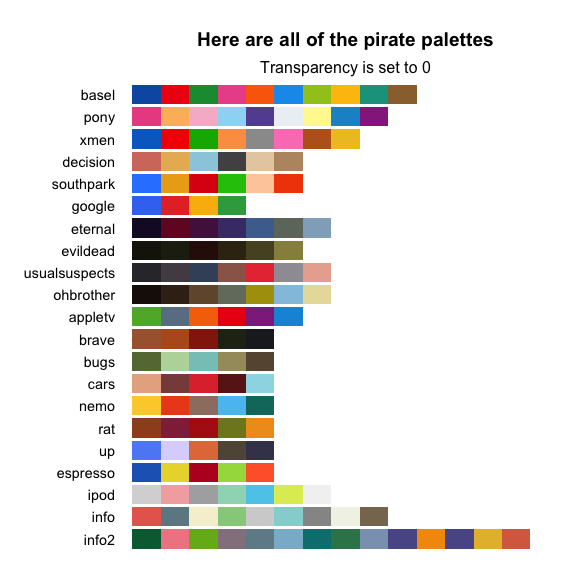Once you find a palette you’d like to use, you can return the colors as a vector by specifying the name of the palette in the palette argument. Here is the Google palette

##        blue         red      yellow       green
## "#3D79F3FF" "#E6352FFF" "#F9B90AFF" "#34A74BFF"

If you want to see a palette in detail (and possibly some images that inspired the palette), include the argument plot.result = T. Here’s where the southpark palette comes from.

piratepal(palette = "southpark",
trans = .5,
plot.result = T)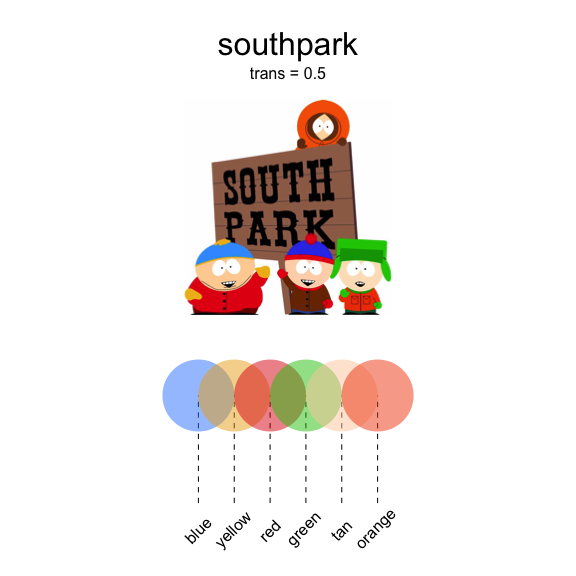You can look at a random palette by setting palette = "random":

piratepal("random", plot.result = T)
## Here's the xmen palette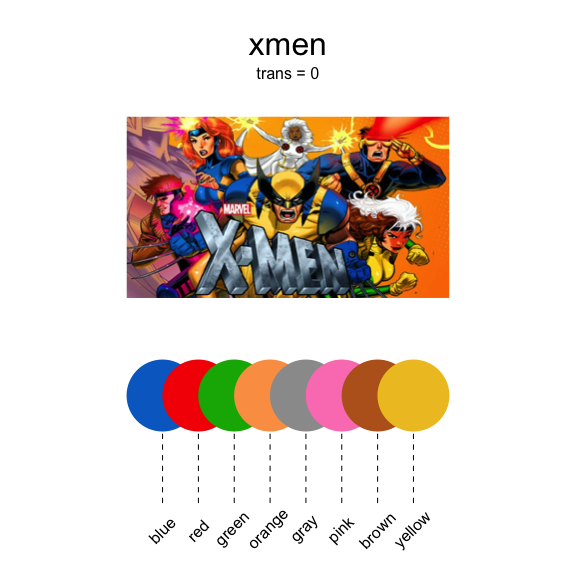Some of the palettes are darker than others. Here’s the nightmare-inducing evildead palette (I’ll up the transparency to .5 to make it a bit less scarring…)

trans = .5,
plot.result = T)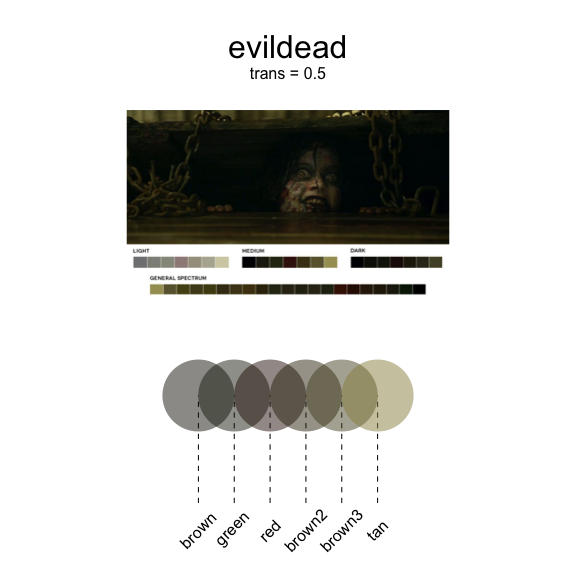Here’s a scatterplot using the xmen palette with piratepal('xmen', trans = .5):

xmen.cols <- piratepal(palette = "xmen",
trans = .5)

x <- rnorm(100)
y <- x + rnorm(100)

plot(x = x, y = y,
col =  xmen.cols,
pch = 16,
cex = 2,
main = "piratepal('xmen', trans = .5)")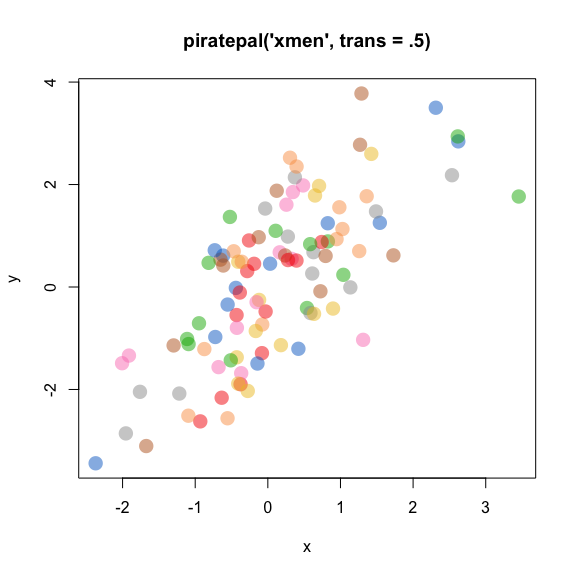Plotting the Up house

Let’s use the basel palette to draw the house from Up Up Trailer

# Set up balloons
balloon.colors <- piratepal("basel", trans = .2)
balloon.x <- rnorm(500, 0)
balloon.y <- rnorm(500, 4, 1)

par(mar = rep(.1, 4))
plot(1, xlim = c(-15, 7), ylim = c(-15, 7),
xlab = "", ylab = "", type = "n",
xaxt = "n", yaxt = "n", bty = "n")

# skyline
start.x <- runif(200, -15, 7)
start.y <- sort(runif(200, -15, -12), decreasing = T)
heights <- runif(200, 2, 4)
widths <- runif(200, .25, 1.5)

rect(start.x, start.y, start.x + widths, start.y + heights,
col = "white", border = gray(.4))

# house
rect(-2, -6, 2, -2)
polygon(c(-2, 0, 2),
c(-2, 0, -2))
rect(-.5, -6, .5, -4)
points(.3, -5)

# strings
line.start.x <- rnorm(500, 0, .2)
line.start.y <- -1 + rnorm(500, 0, .1)
segments(line.start.x,
line.start.y,
balloon.x, balloon.y,
lty = 1, col = gray(.5, .1), lwd = .2)

# balloons
points(balloon.x, balloon.y, pch = 21,
bg = balloon.colors,
col = gray(.9), cex = rnorm(100, 2, .3))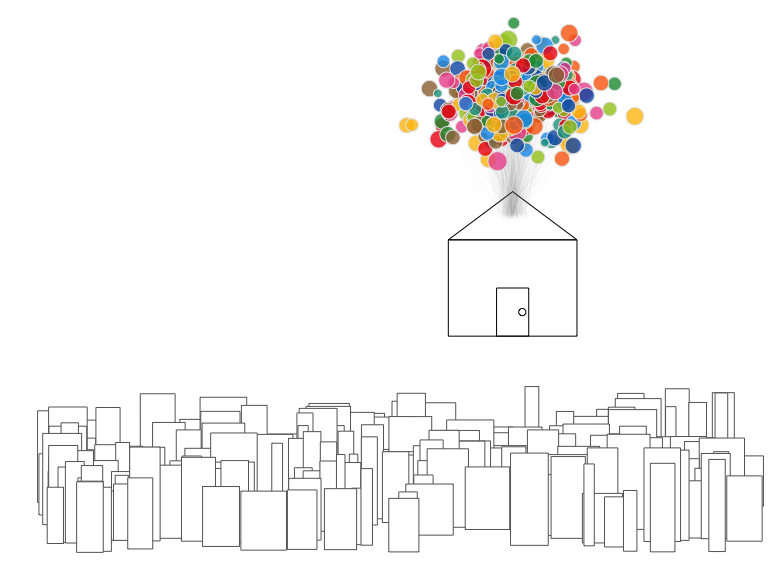Have a favorite palette?

If you have a favorite palette that you’d like me to add, just contact me at yarrr.book@gmail.com and I’ll see what I can do :)